##CS 662 Theory of Parallel Algorithms Speedup

[To Lecture Notes Index]
San Diego State University -- This page last updated February 20, 1996, 1996## Parallel Speedup References

S. G. Akl, M. Cosnard, and A. G. Ferreira, Data-movement-intensive problems: two folk theorems in parallel computation revisited, Theoretical Computer Science 95 (1992) 323-337

G. S. Almasi and A. Gottlieb, Highly Parallel Computing, Benjamin/Cummings, 1989

comp.parallel newsgroup, January 1995

Cosnard and Trystram, Parallel Algorithms and Architectures, International Thompson Computer Press, 1995

## How much Speedup is Possible?

### Amdahl's Law - Serial Bottlenecks

Definitions
Ts(N) = time required by best sequential algorithm to solve a problem of size N
Tp(N) = time required by parallel algorithm using p processors to solve a problem of size N
Sp(N) = Ts(N)/Tp(N)
Sp(N) is the speedup achieved by the parallel algorithm

Amdahl's law

Assume
An operation takes one time unit
A fraction 0 < f < 1 of the operations must be done sequentially
Then Sp(N) <= 1/f

Proof:

We have
f*Ts(N) = number of operations that must be done sequentially
(1-f)*Ts(N) = number of operations that can be done in parallel

We get
Tp(N) = f*Ts(N) + (1-f)*Ts(N)/p
so
Sp(N)
= Ts(N)/(f*Ts(N) + (1-f)*Ts(N)/p)
= 1/(f + (1-f)/p)

But (1-f)/p < 1

Thus Sp(N) <= 1/f
Amdahl's Law with 5% Sequential Operations

Maximum speedup obtainable on an algorithm if 5% of its operations must be done sequentiallyAmdahl's Law Expanded

On many algorithms the fraction of the operations that must be done sequentially is not a constant f, but a function, f(N), of the size of the input of the algorithm .

So the law states
Sp(N) <= 1/f(N)

Example - Sorting N Numbers

Assume
The only operations that must be done sequentially are the reading of the N numbers from disk

Total amount of work for sorting = [[Theta]]( N*lg(N) )

Sequential operations = [[Theta]]( N )

So
f(N) = [[Theta]]( N/{N*lg(N)} ) = [[Theta]]( 1/lg(N) )
``````
Example Multiplying Two N*N Matrices

Two N*N matrices have a total of 2*N*N elements

Assume
The only operations that must be done sequentially are the reading of the 2*N*N numbers from disk

The straight forward method of multiplying two matrices takes
[[Theta]]( N*N*N ) operations

Sequential operations = [[Theta]]( N*N )

So
f(N) = [[Theta]]( N*N/{N*N*N} ) = [[Theta]]( 1/N )

``````
How realistic are these two examples?

Lee's Generalized Amdahl's Law

Let qk be the percentage of the program that can be executed with k processors.

Let t1 be the time to run the program sequentially.

We have: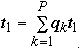and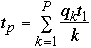SoSetting qk = 1/p w get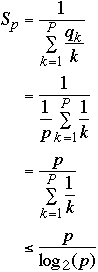So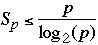Stone's table (1973)
 Speed Up Examples a*p Matrix computations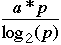Sorts, Linear recursions, polynomial evaluation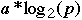Search for an element in a set

### Principle of Unitary Speedup

Lemma 1:
If N processors can perform a computation in one step, then P processors can perform the same computation in ceiling(N/P) steps for 1 <= P <= N

proof:
Each of the N original processors performs one operation
Call that operation I[j ] for j = 1, ..., N
Each of the P processors performs the operation of ceiling(N/P) original processors
So each of the P processors must perform ceiling(N/P) operations
Note the P'th processor may perform fewer operations

Corollary:
If P processors can perform a computation in one step, then one processors can perform the same computation in P steps

Folk Theorem 1. Unitary Speedup
For any algorithm of size N and any number of processors P we have Sp(N) <= P. That is Ep(N) <= 1

proof:
Ts(N) = time required by best sequential algorithm to solve a problem of size N
Tp(N) = time required by parallel algorithm using p processors to solve a problem of size N
Sp(N) = Ts(N)/Tp(N)
Using the corollary a single processor can perform the same operations as the P processors in P*Tp(N) time
So Ts(N) <= P*Tp(N)
Thus Sp(N) <= P*Tp(N) / Tp(N) = P
Folk Theorem 2. (Brent)
If an algorithm involving a total of N operations can be performed in time T on a PRAM with sufficiently many processors, then it can be performed in time T + (N - T)/P on a PRAM with P processors.
proof:
Let Si be the operations performed at time step i on all of the original processors, i = 1, ..., T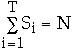Using P processors, the i'th step can be simulated in ceiling(Si /P) time
But ceiling(Si /P) <= (Si /P) + (P - 1)/P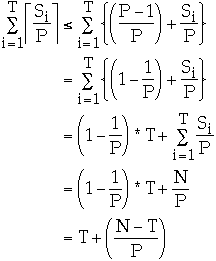Uses of Brent's Theorem
• Simplify analysis
• Justify algorithms with large number of processors
• Produce optimal parallel algorithms
It is possible to improve a parallel algorithm by using few processors

Adding N integers with P = N/2 processors

Assume that N is a power of 2
```J = N/2
while J >= 1 do
for K = 1 to J do in Parallel
Processor K: A[K] = A[2K-1]+A[2K]
end for
J = J/2
end while
```

Time Complexity [[Theta]]( lg(N) )

Cost [[Theta]]( N*Lg(N) )

Let P = ceiling( N/lg(N) ) be the number of processors

```for I = 1 to P do in Parallel
Processor I:
B[I] = 0;
for K = 1 to lg(N) do
B[I] = A[{(I-1)*N/P}+K] + B[I]
end for
end for

J = ceiling(N/[2*lg(N)])

while J >= 1 do
for K = 1 to J do in Parallel
Processor K: B[K] = B[2K-1]+B[2K]
end for
J = J/2
end while
```

Time Complexity [[Theta]](lg(N)) + [[Theta]]( lg[N/lg(N)] )= [[Theta]]( lg(N) )

Cost [[Theta]]( Lg(N) * N/lg(N) ) = [[Theta]]( N )

### Superlinear Speedup in Theory

Problem: Messy List
We are given P distinct integers I1, I2, ..., IP such that Ik <= P for all k.
Note Ik can be negative.
The integers are stored in an array A, such that A[K] = Ik
Modify A so that for 1 <= K <= P we have:
A[Ik] = Ik if and only if 1 <= Ik <= P
A[K] = Ik otherwise

Example

Let P = 4
```					A	A	A	A

Original values	4		1		-2		3

Modified values	1		1		3		4
```
Parallel Solution
```for I = 1 to P do in Parallel
Processor I: A[A[I]] := A[I]
```

Time required: 1 time unit

Sequential Solution

Theorem.
The problem Messy List cannot be solved in less than 2P -1 time units using the RAM model.

proof:

At some point the solution will perform an operation like:
Read X; if X > 0 then A[X] = X
(1)
Consider the first time we perform such an operation
Since we overwrite A[X] in line (1) we need to save old value of A[X] before we do line (1)
But X can be any index between 1 and P so we need to save P -1 elements of A before we perform (1)
We also need to perform (1) P times.

The speedup is 2P -1

### Superlinear Speedup in Practice

Superlinear speedups are found in practice

All examples that I know of are due to the additional resources used in the parallel code over that used in the sequential code

Cache effects are a common cause of superlinear speedups

The following example is from comp.parallel Jan. 1995

Newsgroups: comp.parallel
Subject: Help - explain superlinear speedup?
From: slater@nuc.berkeley.edu (Steve Slater)
I have a program which has superlinear speedup and I can't explain it. Does anyone have any ideas. Here is the summary.
I am using a code which passes messages using p4, on 4 Sparc 2's running SunOS 4.1.3. The code solves coupled matrix equations, much like a heat equation. The processors are each assigned a geometrical region like:
```	------------------
|        |       |
|   A    |   B   |
|________|_______|
|        |       |
|   C    |   D   |
|________|_______|
```
Each job/process analyzes only one region of A through D.
What happens in the code (not really important to my problem though) is a matrix is solved for each A-D, then the boundary conditions are passed between each region (outgoing heat current = incoming for each neighbor) and the matrix equations are solved locally again. The process repeats until the solution converges.
With p4, I first run 4 processes (4 regions) on only 1 machine. The messages are passing through sockets. Then I run on 2 machines, each having 2 processes (2 regions), and finally on 4 machines, each having 1 process (region).
You would expect less than linear speedup since with only one machine, no messages are sent over the ethernet, they are just communicated via sockets. But I get very superlinear speedup like:
1 proc:556 sec 4 unique processes on 1 machine
2 proc:204 sec 2 processes on each of 2 machines
4 proc: 38 sec 1 process on each machine
There was NO memory swapping occurring during the entire execution time. I would periodically check with ps.
Does anyone have any thoughts?

Steve Slater
slater@nuc.berkeley.edu

From: Krste Asanovic <krste@icsi.berkeley.edu>
Subject: Re: Help - explain superlinear speedup?
There are two possible cache effects. The first is that each Sparc-2 only has 64KB of unified cache. If your data set + code fits into 64KB you'll see a marking improvement over the case when it doesn't.
The second is the limited TLB size. I don't have the Sparc-2 MMU numbers handy, but I think it supported 64 entries for 4KB pages, i.e. 256KB mapped simultaneously at most. If a single process's code + data fits into the TLB, you'll see a marked difference.
These differences are exaggerated if your code makes repeated sweeps over these data regions.
--
Krste Asanovic email: krste@icsi.berkeley.edu

Newsgroups: comp.parallel